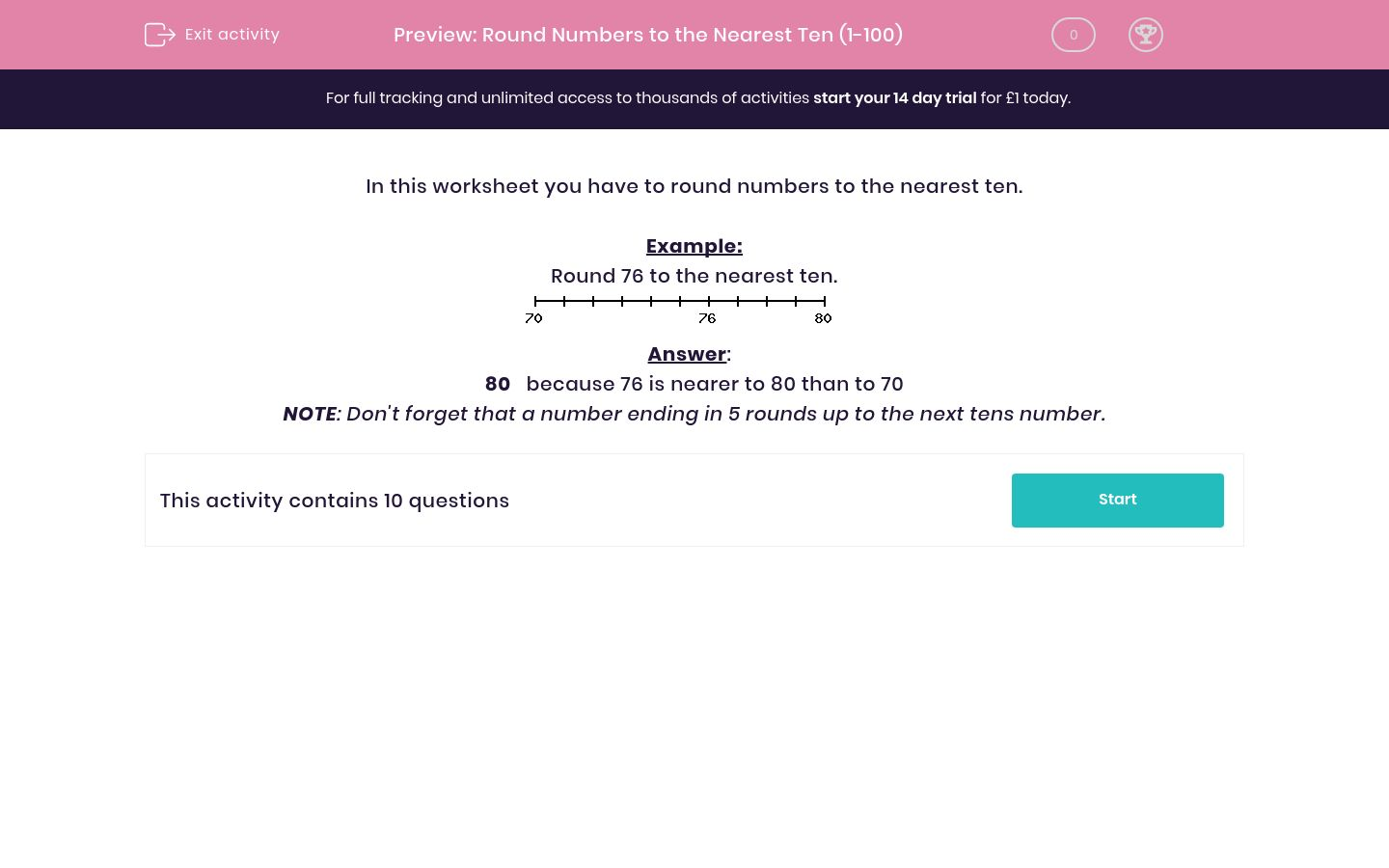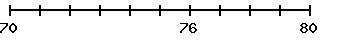# Round Numbers to the Nearest Ten (1-100)

In this worksheet, students round numbers to the nearest ten.Key stage:  KS 2

Curriculum topic:   Maths and Numerical Reasoning

Curriculum subtopic:   Place Value

Difficulty level:### QUESTION 1 of 10

In this worksheet you have to round numbers to the nearest ten.

Example:

Round 76 to the nearest ten.80   because 76 is nearer to 80 than to 70

NOTE: Don't forget that a number ending in 5 rounds up to the next tens number.

82 rounded to the nearest ten is ?

88 rounded to the nearest ten is ?

55 rounded to the nearest ten is ?

36 rounded to the nearest ten is ?

17 rounded to the nearest ten is ?

59 rounded to the nearest ten is ?

13 rounded to the nearest ten is ?

44 rounded to the nearest ten is ?

53 rounded to the nearest ten is ?

23 rounded to the nearest ten is ?

• Question 1

82 rounded to the nearest ten is ?

80
• Question 2

88 rounded to the nearest ten is ?

90
• Question 3

55 rounded to the nearest ten is ?

60
• Question 4

36 rounded to the nearest ten is ?

40
• Question 5

17 rounded to the nearest ten is ?

20
• Question 6

59 rounded to the nearest ten is ?

60
• Question 7

13 rounded to the nearest ten is ?

10
• Question 8

44 rounded to the nearest ten is ?

40
• Question 9

53 rounded to the nearest ten is ?

50
• Question 10

23 rounded to the nearest ten is ?

20
---- OR ----

Sign up for a £1 trial so you can track and measure your child's progress on this activity.

### What is EdPlace?

We're your National Curriculum aligned online education content provider helping each child succeed in English, maths and science from year 1 to GCSE. With an EdPlace account you’ll be able to track and measure progress, helping each child achieve their best. We build confidence and attainment by personalising each child’s learning at a level that suits them.

Get started